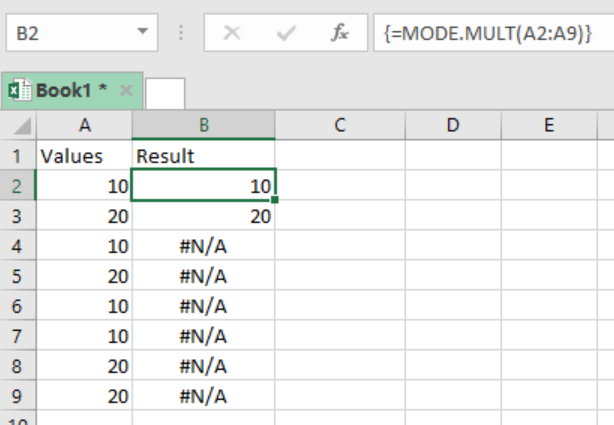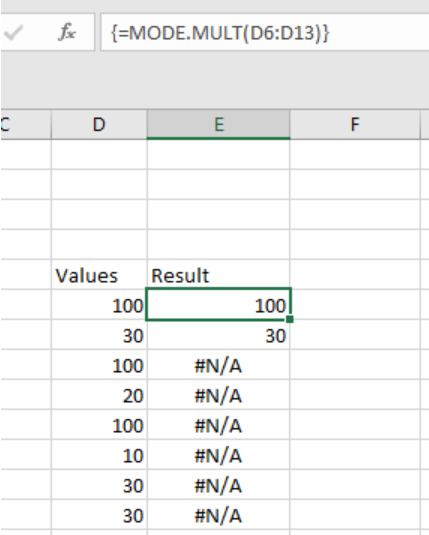Get instant live expert help with Excel or Google Sheets“My Excelchat expert helped me in less than 20 minutes, saving me what would have been 5 hours of work!”

#### Post your problem and you’ll get Expert help in seconds.

Your message must be at least 40 characters
Our professional Expert are available now. Your privacy is guaranteed.

# Excel MODE.MULT Function

If we want to get the most occurring numbers in an excel set in a vertical array, we can deploy the excel MODE.MULT function. Here is an elaborative guide on how to go about it.Figure 1: Using MODE.MULT function

## General syntax of the formula

`=MODE.MULT (number1, [number2], …)`

Where;

• Number1– refers to the number or cell reference containing numeric values
• Number2– number or cell reference with numeric values, it’s optional

## Understanding the formula

• This function will return a vertical array of those values that are most occurring in your dataset.
• One can supply the numbers in various forms, either as numbers, named ranges or even cell reference with the numeric values.
• This function can only accept arguments to a maximum of 254.
• The formula should be entered in an array form, i.e. Control + Shift + Enter
• You can use the TRANSPOSE function to get the values in a horizontal array. All you will need to do is to transpose the original vertical array. This can be done by the following formula; `=TRANSPOSE(MODE.MULT(range))`

## Note

The MODE function will ignore empty cells, cells with text or those with Boolean values.

Also, the function will return a #N/A error if the supplied number do not have duplicates.

Here, we want to know those numbers that are most occurring. To do this, we proceed as follows;

Step 1: Fill your values in column D as shown above.

Step 2: Select the vertical cells you want to get your results in

Step 3: In cell E6, put the formula; `=MODE.MULT (D6:D13)`

Step 4: Press Control + Shift + Enter

## ExampleFigure 2: Finding most frequent values with excel MODE.MULT function

We can see in the above example, the formula results in 100 and 30 because those are the most frequent numbers of the dataset in column D.

## Instant Connection to an Expert through our Excelchat Service

Most of the time, the problem you will need to solve will be more complex than a simple application of a formula or function. If you want to save hours of research and frustration, try our live Excelchat service! Our Excel Experts are available 24/7 to answer any Excel question you may have. We guarantee a connection within 30 seconds and a customized solution within 20 minutes.

Solution examplesi want to hide a column but still use it in formula. is this even possible?
Solved by X. E. in 11 minsC5 contains 1625MPGW, F5 contains quantity. Need to search for MPGW and have quantity insert into column H5.
Solved by E. C. in 33 minsNeed help with lookup when you have multiple lookup values
Solved by V. J. in 20 minsI have a spread sheet with Items and sub Items. I would like to know if I can place a letter in another column it can get it to list the sub item number on another page in order
Solved by S. H. in 60 minsNeed to seperate text thats in a cell so i can sum them
Solved by G. C. in 10 mins